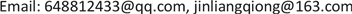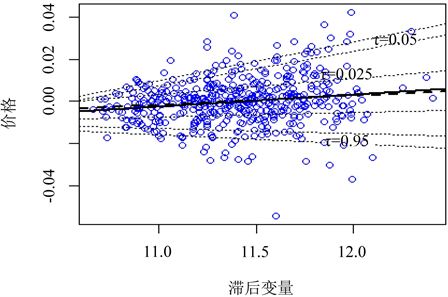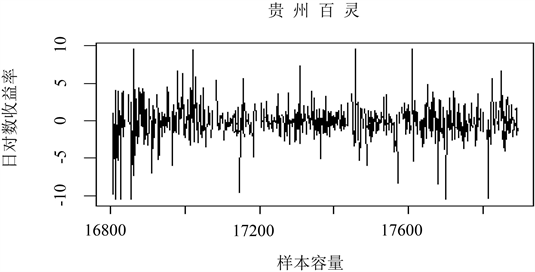﻿ 基于分位数回归模型的VaR研究——以贵州百灵股票为例 VaR Research Based on Quantile Regression Model—Taking Guizhou Bailing Stock as an Example

Statistics and Application
Vol. 08  No. 02 ( 2019 ), Article ID: 29913 , 6 pages
10.12677/SA.2019.82040

VaR Research Based on Quantile Regression Model

—Taking Guizhou Bailing Stock as an Example

Shitong Fu, Liangqiong Jin

Institute of Data Science and Information Engineering, Guizhou Minzu University, Guiyang GuizhouReceived: Apr. 4th, 2019; accepted: Apr. 19th, 2019; published: Apr. 26th, 2019ABSTRACT

Taking Guizhou Bailing as the research object, this paper established the VaR model of Quantile Regression to describe the risk measurement of stock data. The Quantile Regression model and the traditional GARCH model were compared with the risk measurement results of VaR. The empirical results show that the Quantile Regression method achieves relatively optimistic results in the data samples, and the accuracy of the results based on the Quantile Regression model is higher than that of the traditional model.

Keywords:Value-at-Risk, Quantile Regression Method, Garch Model

——以贵州百灵股票为例Copyright © 2019 by authors and Hans Publishers Inc.

This work is licensed under the Creative Commons Attribution International License (CC BY).

http://creativecommons.org/licenses/by/4.0/1. 引言

2. 基于分位数回归模型的VaR风险测度

$Y$ 为一元随机变量，其右连续分布函数 $F\left(y\right)={P}_{r}\left(Y\le y\right)$$\forall 0<\tau <1$ ，得到 $Y$ 的第 $\tau$ 位分位数表示为：

${F}^{-1}\left(\tau \right)=\mathrm{inf}\left\{y|F\left(y\ge \tau \right)\right\}$ (1)

$X$$p$ 维回归变量，当 $X=x$ 时，定义 $Y$ 的条件分布为：

${F}_{Y}^{-1}\left(\tau |x\right)={x}^{T}\beta \left(\tau \right)$ (2)

$\underset{\beta }{\mathrm{min}}\underset{t=1}{\overset{n}{\sum }}{\rho }_{\tau }\left({y}_{t}-{x}_{t}^{T}\beta \right)$ (3)

${\rho }_{\left(\tau \right)}\left(u\right)=\left\{\begin{array}{l}\tau u,u\ge 0\\ \left(1-\tau \right)u,u<0\end{array}$ (4)

${Q}_{{y}_{t}}\left(\tau |{x}_{i}\right)=\mathrm{inf}\left\{y\in R:F\left(y|x\right)\ge \tau \right\}\equiv {F}^{-1}\left(\tau |x\right)$ (5)

${Q}_{{y}_{t}}\left(\tau |{x}_{i}\right)={x}^{T}\beta \left(\tau \right)$ (6)

$Va{R}_{\tau }={Q}_{{y}_{t}}\left(\tau |{x}_{i}\right)$ (7)

${Q}_{{y}_{t}}\left(\tau |{y}_{t-1},{y}_{t-2},\cdots ,{y}_{t-m}\right)={\alpha }_{0}+{\alpha }_{1}{y}_{t-1}+{y}_{t-2}+\cdots \text{+}{\alpha }_{m}{y}_{t-m}$ (8)

3. 基于GARCH模型的VaR风险测度

$\left\{\begin{array}{l}{y}_{t}={{X}^{\prime }}_{t}+{\epsilon }_{t},{\epsilon }_{t}={\sigma }_{t}{\eta }_{t}\\ {\sigma }_{t}^{2}=\omega +\underset{i=1}{\overset{q}{\sum }}{\alpha }_{i}{\epsilon }_{t-i}^{2}+\underset{i=1}{\overset{p}{\sum }}{\beta }_{i}{\sigma }_{t-i}^{2}\end{array}$ (9)

${\sigma }_{t}^{2}=\omega +{\alpha }_{1}{\epsilon }_{t-1}^{2}+{\beta }_{1}{\sigma }_{t-1}^{2}$ (10)

$k=\frac{E\left({\epsilon }_{t}^{2}\right)}{{\left[E\left({\epsilon }_{t}^{2}\right)\right]}^{2}}=\frac{3\left[1-{\left({\alpha }_{1}+{\beta }_{1}\right)}^{2}\right]}{1-2{\alpha }_{1}^{2}-{\left({\alpha }_{1}+{\beta }_{1}\right)}^{2}}>3$ (11)

${y}_{t}={\varphi }_{0}+\underset{i=1}{\overset{m}{\sum }}{\varphi }_{i}{y}_{t-i}+{a}_{t}-\underset{j=1}{\overset{n}{\sum }}{\theta }_{j}{a}_{t-j},{a}_{t}={\sigma }_{t}{\epsilon }_{t}$ (12)

${\sigma }_{t}^{2}=\omega +\underset{i=1}{\overset{p}{\sum }}{\alpha }_{i}{a}_{t-i}^{2}+\underset{j=1}{\overset{q}{\sum }}{\beta }_{j}{\sigma }_{t-j}^{2}$ (13)

${\stackrel{^}{y}}_{t}\left(1\right)={\varphi }_{0}+\underset{i=1}{\overset{m}{\sum }}{\varphi }_{i}{y}_{t+1-i}-\underset{j=1}{\overset{n}{\sum }}{\theta }_{j}{a}_{t+1-j}$ (14)

${\stackrel{^}{\sigma }}_{t}^{2}\left(1\right)=\omega +\underset{i=1}{\overset{p}{\sum }}{\alpha }_{i}{a}_{t+1-i}^{2}-\underset{j=1}{\overset{q}{\sum }}{\beta }_{j}{\sigma }_{t+1-j}^{2}$ (15)

${\text{VaR}}_{1-\tau }={\stackrel{^}{y}}_{t}\left(1\right)+\frac{{t}_{1-\tau }\left(s\right)}{\sqrt{s/\left(s-2\right)}}\stackrel{^}{\sigma }\left(1\right)$ (16)

4. 实证研究

4.1. 分位数回归的VaR模型

 $Q\left(\tau |{y}_{t-i}\right)=3.8070756+0.25801940{y}_{t-1}+0.32799306{y}_{t-2}+0.0950663{y}_{t-3}$ (17)

4.2. GARCH的VaR模型Figure 1. Quantile regression modelFigure 2. Time series diagramTable 1. The result of the basic characteristic of logarithmic rate of return

$\left\{\begin{array}{l}{r}_{t}={\sigma }_{t}{\epsilon }_{t}\text{},{\epsilon }_{t}~\left(4.8670\right)\\ {\sigma }_{t}^{2}=0.12977+0.07301{\epsilon }_{t-1}^{2}+0.9154{\sigma }_{t-1}^{2}\end{array}$ (18)

5. 总结

VaR已然成为当今国际上最为主流的一种有效的金融风险度量的工具，被金融各界人士广泛运用。文章基于分位数回归模型，对贵州百灵股票的VaR进行测度研究，通过Kupiec似然比检验将模型一与GARCH模型作了对比，得出GARCH模型失败率要高于模型一，故分位数回归模型在对VaR的风险测度研究上要比GARCH模型略稳定。当然由于金融数据本身的特点过于复杂，也意味着金融风险测度不会是一个简单的问题。虽然它的计算方法随着研究人员们的不断努力在不停地改善着，但时至今日，大部分的方法、模型也都是为VaR的测度研究提供参考。

VaR Research Based on Quantile Regression Model—Taking Guizhou Bailing Stock as an Example[J]. 统计学与应用, 2019, 08(02): 364-369. https://doi.org/10.12677/SA.2019.82040

1. 1. 张晨. VaR模型在我国金融风险管理中的运用研究[J]. 合肥工业大学学报:自然科学版, 2003, 26(3): 441-445.

2. 2. 关静, 史道济. 分位数回归与上证综指VaR研究[J]. 统计与信息论坛, 2008, 23(12): 15-19.

3. 3. Konenker, R. and Bassett, G. (1978) Regression Quantiles. Econometrica, 46, 33-50. https://doi.org/10.2307/1913643

4. 4. 许启发, 徐金菊, 蒋翠侠. 基于神经网络分位数回归的多期CVaR风险测度[J]. 数理统计与管理, 2017, 36(4): 715-730.

5. 5. 李治章, 王帅. 互联网金融对中国商业银行系统性风险溢出效应的测度——基于GARCH-CoVaR模型的研究[J].经济研究导刊, 2018(36): 50-53.

6. 6. 许启发, 张金秀, 蒋翠侠. 基于非线性分位数回归模型的多期VaR风险测度[J]. 中国管理科学, 2015, 23(3): 56-65.